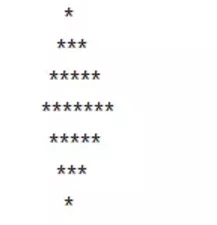### 值得收藏的30道Python练手题（附详解）

1. 已知一个字符串为 “hello_world_yoyo”，如何得到一个队列 [“hello”,”world”,”yoyo”] ？

``test = 'hello_world_yoyo'print(test.split("_"))12``

``['hello', 'world', 'yoyo']``

2. 有个列表 [“hello”, “world”, “yoyo”]，如何把列表里面的字符串联起来，得到字符串 “hello_world_yoyo”？

``test = ["hello", "world", "yoyo"]print("_".join(test))``

``hello_world_yoyo``

for 循环拼接如下：

``test = ["hello", "world", "yoyo"]# 定义一个空字符串j = ''# 通过 for 循环打印出列表中的数据for i in test:    j = j + "_" + i# 因为通过上面的字符串拼接，得到的数据是“_hello_world_yoyo”，前面会多一个下划线_，所以把这个下划线去掉print(j.lstrip("_"))``

3. 把字符串 s 中的每个空格替换成”%20”，输入：s = “We are happy.”，输出：“We%20are%20happy.”。

``s = 'We are happy.'print(s.replace(' ', '%20'))12``

``We%20are%20happy.``

4. Python 如何打印 99 乘法表？

for 循环打印：

``for i in range(1, 10):    for j in range(1, i+1):        print('{}x{}={}\t'.format(j, i, i*j), end='')    print()``

while 循环实现：

``i = 1while i <= 9:    j = 1    while j <= i:        print("%d*%d=%-2d"%(i,j,i*j),end = ' ')  # %d： 整数的占位符，'-2'代表靠左对齐，两个占位符        j += 1    print()    i += 1``

``1x1=1 1x2=2 2x2=4 1x3=3 2x3=6 3x3=9 1x4=4 2x4=8 3x4=12 4x4=16 1x5=5 2x5=10 3x5=15 4x5=20 5x5=25 1x6=6 2x6=12 3x6=18 4x6=24 5x6=30 6x6=36 1x7=7 2x7=14 3x7=21 4x7=28 5x7=35 6x7=42 7x7=49 1x8=8 2x8=16 3x8=24 4x8=32 5x8=40 6x8=48 7x8=56 8x8=64 1x9=9 2x9=18 3x9=27 4x9=36 5x9=45 6x9=54 7x9=63 8x9=72 9x9=81``

5. 从下标 0 开始索引，找出单词 “welcome” 在字符串“Hello, welcome to my world.” 中出现的位置，找不到返回 -1。

``def test():    message = 'Hello, welcome to my world.'    world = 'welcome'    if world in message:        return message.find(world)    else:        return -1  print(test())  结果：7``

6. 统计字符串“Hello, welcome to my world.” 中字母 w 出现的次数。

``def test():    message = 'Hello, welcome to my world.'    # 计数    num = 0    # for 循环 message    for i in message:        # 判断如果 ‘w’ 字符串在 message 中，则 num +1        if 'w' in i:            num += 1    return num  print(test()) # 结果2``

7. 输入一个字符串 str，输出第 m 个只出现过 n 次的字符，如在字符串 gbgkkdehh 中，找出第 2 个只出现 1 次的字符，输出结果：d

``def test(str_test, num, counts):    """    :param str_test: 字符串    :param num: 字符串出现的次数    :param count: 字符串第几次出现的次数    :return:    """    # 定义一个空数组，存放逻辑处理后的数据    list = []     # for循环字符串的数据    for i in str_test:        # 使用 count 函数，统计出所有字符串出现的次数        count = str_test.count(i, 0, len(str_test))         # 判断字符串出现的次数与设置的counts的次数相同，则将数据存放在list数组中        if count == num:            list.append(i)     # 返回第n次出现的字符串    return list[counts-1]  print(test('gbgkkdehh', 1, 2)) 结果：d``

8. 判断字符串 a = “welcome to my world” 是否包含单词 b = “world”，包含返回 True，不包含返回 False。

``def test():    message = 'welcome to my world'    world = 'world'     if world in message:        return True    return False  print(test()) 结果：True``

9. 从 0 开始计数，输出指定字符串 A = “hello” 在字符串 B = “hi how are you hello world, hello yoyo!”中第一次出现的位置，如果 B 中不包含 A，则输出 -1。

``def test():    message = 'hi how are you hello world, hello yoyo!'    world = 'hello'     return message.find(world)  print(test()) 结果：15``

10. 从 0 开始计数，输出指定字符串 A = “hello”在字符串 B = “hi how are you hello world, hello yoyo!”中最后出现的位置，如果 B 中不包含 A，则输出 -1。

``def test(string, str):    # 定义 last_position 初始值为 -1    last_position = -1    while True:        position = string.find(str, last_position+1)        if position == -1:            return last_position        last_position = position  print(test('hi how are you hello world, hello yoyo!', 'hello')) 结果：28``

11. 给定一个数 a，判断一个数字是否为奇数或偶数。

``while True:    try:        # 判断输入是否为整数        num = int(input('输入一个整数：'))    # 不是纯数字需要重新输入    except ValueError:         print("输入的不是整数！")        continue    if num % 2 == 0:        print('偶数')    else:        print('奇数')    break 结果：输入一个整数：100偶数``

12. 输入一个姓名，判断是否姓王。

``def test():    user_input = input("请输入您的姓名：")     if user_input == '王':        return "用户姓王"     return "用户不姓王" print(test()) 结果：请输入您的姓名：王总用户姓王``

13. 如何判断一个字符串是不是纯数字组成？

``def test(num):    try:        return float(num)    except ValueError:        return "请输入数字"  print(test('133w3'))``

14. 将字符串 a = “This is string example….wow!” 全部转成大写，字符串 b = “Welcome To My World” 全部转成小写。

``a = 'This is string example….wow!'b = 'Welcome To My World' print(a.upper())print(b.lower())``

15. 将字符串 a = “ welcome to my world ”首尾空格去掉

Python 提供了strip() 方法，可以去除首尾空格，rstrip() 去掉尾部空格，lstrip() 去掉首部空格，replace(” “, “”) 去掉全部空格。

``a = '  welcome to my world   'print(a.strip())``

``def trim(s):    flag = 0    if s[:1]==' ':        s = s[1:]        flag = 1    if s[-1:] == ' ':        s = s[:-1]        flag = 1    if flag==1:        return    trim(s)    else:        return sprint(trim('  Hello world!  '))``

``def trim(s):    while(True):        flag = 0        if s[:1]==' ':            s = s[1:]            flag = 1        if s[-1:] == ' ':            s = s[:-1]            flag = 1        if flag==0:            break    return sprint(trim('  Hello world!  '))``

``def test():    s = 'ajldjlajfdljfddd'    # 定义一个数组存放数据    str_list = []    # for循环s字符串中的数据，然后将数据加入数组中    for i in s:        # 判断如果数组中已经存在这个字符串，则将字符串移除，加入新的字符串        if i in str_list:            str_list.remove(i)         str_list.append(i)    # 使用 sorted 方法，对字母进行排序    a = sorted(str_list)    # sorted方法返回的是一个列表，这边将列表数据转换成字符串    return "".join(a)  print(test()) 结果：adfjl``

17. 打印出如下图案（菱形）:``def test():    n = 8    for i in range(-int(n/2), int(n/2) + 1):        print(" "*abs(i), "*"*abs(n-abs(i)*2))  print(test()) 结果：    **   ****  ****** ********  ******   ****    **``

18.  给一个不多于 5 位的正整数（如 a = 12346），求它是几位数和逆序打印出各位数字。

``class Test:     # 计算数字的位数    def test_num(self, num):        try:            # 定义一个 length 的变量，来计算数字的长度            length = 0            while num != 0:                # 判断当 num 不为 0 的时候，则每次都除以10取整                length += 1                num = int(num) // 10            if length > 5:                return "请输入正确的数字"            return length        except ValueError:            return "请输入正确的数字"     # 逆序打印出个位数    def test_sorted(self, num):        if self.test_num(num) != "请输入正确的数字":            # 逆序打印出数字            sorted_num = num[::-1]            # 返回逆序的个位数            return sorted_num[-1] print(Test().test_sorted('12346')) 结果：1``

19. 如果一个 3 位数等于其各位数字的立方和，则称这个数为水仙花数。例如：153 = 13 + 53 + 33，因此 153 就是一个水仙花数。那么如何求 1000 以内的水仙花数（3 位数）。

``def test():    for num in range(100, 1000):        i = num // 100        j = num // 10 % 10        k = num % 10        if i ** 3 + j ** 3 + k ** 3 == num:            print(str(num) + "是水仙花数") test()``

20. 求 1+2+3…+100 相加的和。

``i = 1for j in range(101):    i = j + i print(i) 结果：5051``

21. 计算 1-2+3-4+5-…-100 的值。

``def test(sum_to):        # 定义一个初始值    sum_all = 0    # 循环想要计算的数据    for i in range(1, sum_to + 1):        sum_all += i * (-1) ** (1 + i)    return sum_all  if __name__ == '__main__':    result = test(sum_to=100)    print(result) -50``

22. 现有计算公式 13 + 23 + 33 + 43 + …….+ n3，如何实现：当输入 n = 5 时，输出 225（对应的公式 : 13 + 23 + 33 + 43 + 53 = 225）。

``def test(n):    sum = 0    for i in range(1, n+1):        sum += i*10+i    return sum print(test(5)) 结果：225``

23. 已知 a 的值为“hello”，b 的值为“world”，如何交换 a 和 b 的值，得到 a 的值为“world”，b 的值为”hello”？

``a = 'hello'b = 'world' c = aa = bb = cprint(a, b)``

24. 如何判断一个数组是对称数组？

``def test():    x = [1, 'a', 0, '2', 0, 'a', 1]    # 通过下标的形式，将字符串逆序进行比对    if x == x[::-1]:        return True    return False print(test()) 结果：True``

25. 如果有一个列表 a = [1,3,5,7,11]，那么如何让它反转成 [11,7,5,3,1]，并且取到奇数位值的数字 [1,5,11]？

``def test():    a = [1, 3, 5, 7, 11]    # 逆序打印数组中的数据    print(a[::-1])    # 定义一个计数的变量    count = 0    for i in a:        # 判断每循环列表中的一个数据，则计数器中会 +1        count += 1        # 如果计数器为奇数，则打印出来        if count % 2 != 0:            print(i)  test() 结果：[11, 7, 5, 3, 1]1511``

26. 对列表 a = [1, 6, 8, 11, 9, 1, 8, 6, 8, 7, 8] 中的数字从小到大排序。

``a = [1, 6, 8, 11, 9, 1, 8, 6, 8, 7, 8]print(sorted(a)) 结果：[1, 1, 6, 6, 7, 8, 8, 8, 8, 9, 11]``

27. 找出列表 L1 = [1, 2, 3, 11, 2, 5, 3, 2, 5, 33, 88] 中最大值和最小值。

``L1 = [1, 2, 3, 11, 2, 5, 3, 2, 5, 33, 88]print(max(L1))print(min(L1)) 结果：881``

``class Test(object):     def __init__(self):        # 测试的列表数据        self.L1 = [1, 2, 3, 11, 2, 5, 3, 2, 5, 33, 88]         # 从列表中取第一个值，对于数据大小比对        self.num = self.L1     def test_small_num(self, count):        """        :param count: count为 1，则表示计算最大值，为 2 时，表示最小值        :return:        """        # for 循环查询列表中的数据        for i in self.L1:            if count == 1:                # 循环判断当数组中的数据比初始值小，则将初始值替换                if i > self.num:                    self.num = i                        elif count == 2:                if i < self.num:                    self.num = i                                elif count != 1 or count != 2:                return "请输入正确的数据"         return self.num  print(Test().test_small_num(1))print(Test().test_small_num(2))结果：881``

28. 找出列表 a = [“hello”, “world”, “yoyo”, “congratulations”] 中单词最长的一个。

``def test():    a = ["hello", "world", "yoyo", "congratulations"]        # 统计数组中第一个值的长度    length = len(a)        for i in a:        # 循环数组中的数据，当数组中的数据比初始值length中的值长，则替换掉length的默认值        if len(i) > length:            length = i    return length  print(test()) 结果：congratulations``

29. 取出列表 L1 = [1, 2, 3, 11, 2, 5, 3, 2, 5, 33, 88] 中最大的三个值。

``def test():    L1 = [1, 2, 3, 11, 2, 5, 3, 2, 5, 33, 88]    return sorted(L1)[:3] print(test()) 结果：[1, 2, 2]``

30. 把列表 a = [1, -6, 2, -5, 9, 4, 20, -3] 中的数字绝对值。

``def test():    a = [1, -6, 2, -5, 9, 4, 20, -3]    # 定义一个数组，存放处理后的绝对值数据    lists = []    for i in a:     # 使用 abs() 方法处理绝对值        lists.append(abs(i))    return lists print(test()) 结果：[1, 6, 2, 5, 9, 4, 20, 3]``

``推荐阅读:入门: 最全的零基础学Python的问题  | 零基础学了8个月的Python  | 实战项目 |学Python就是这条捷径干货:爬取豆瓣短评，电影《后来的我们》 | 38年NBA最佳球员分析 |   从万众期待到口碑扑街！唐探3令人失望  | 笑看新倚天屠龙记 | 灯谜答题王 ｜用Python做个海量小姐姐素描图 ｜碟中谍这么火，我用机器学习做个迷你推荐系统电影趣味:弹球游戏  | 九宫格  | 漂亮的花 | 两百行Python《天天酷跑》游戏!AI: 会做诗的机器人 | 给图片上色 | 预测收入 | 碟中谍这么火，我用机器学习做个迷你推荐系统电影小工具: Pdf转Word，轻松搞定表格和水印！ | 一键把html网页保存为pdf！|  再见PDF提取收费！ | 用90行代码打造最强PDF转换器，word、PPT、excel、markdown、html一键转换 | 制作一款钉钉低价机票提示器！ ｜60行代码做了一个语音壁纸切换器天天看小姐姐！｜``

• 1).卧槽！Pdf转Word用Python轻松搞定！

• 2).学Python真香！我用100行代码做了个网站，帮人PS旅行图片，赚个鸡腿吃

• 3).首播过亿，火爆全网，我分析了《乘风破浪的姐姐》，发现了这些秘密

• 4).80行代码！用Python做一个哆来A梦分身

• 5).你必须掌握的20个python代码，短小精悍，用处无穷

• 6).30个Python奇淫技巧集

• 7).我总结的80页《菜鸟学Python精选干货.pdf》,都是干货

• 8).再见Python！我要学Go了！2500字深度分析！

• 9).发现一个舔狗福利！这个Python爬虫神器太爽了，自动下载妹子图片